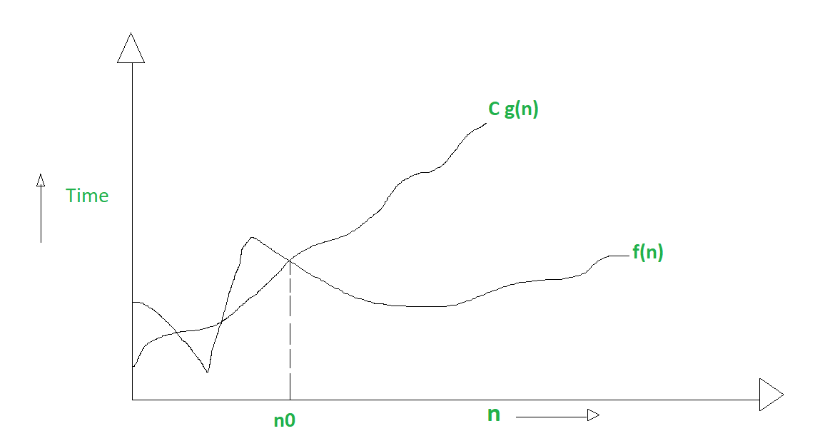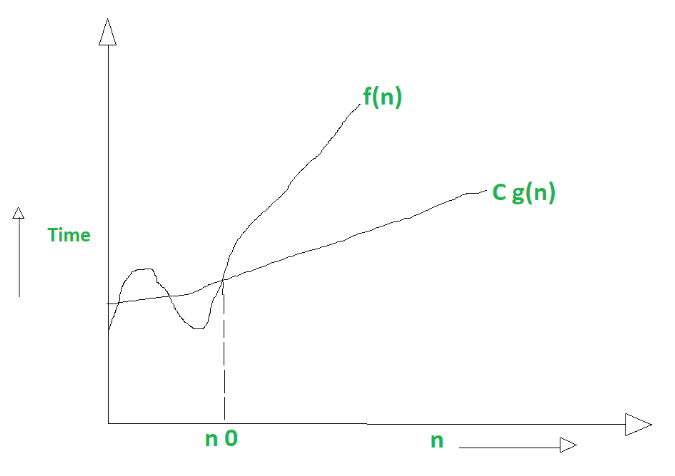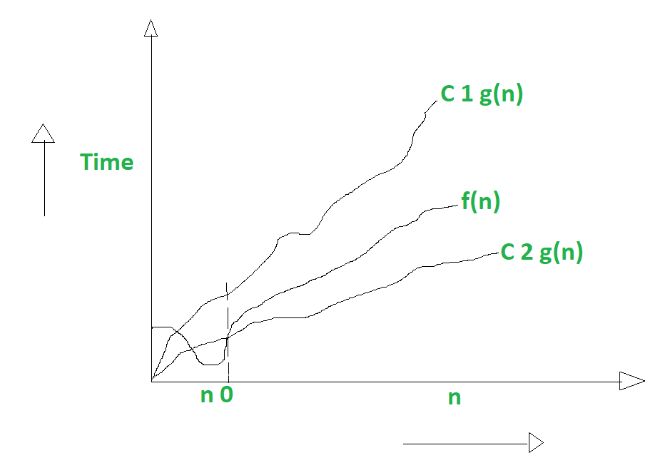# Difference between Big Oh, Big Omega and Big Theta

• Difficulty Level : Easy
• Last Updated : 13 Jul, 2022

Prerequisite – Asymptotic Notations, Properties of Asymptotic Notations, Analysis of Algorithms
1. Big oh notation (O):

It is define as upper bound and upper bound on an algorithm is the most amount of time required ( the worst case performance).
Big oh notation is used to describe asymptotic upper bound

Mathematically, if f(n) describes the running time of an algorithm; f(n) is O(g(n)) if there exist positive constant C and n0 such that,

0 <= f(n) <= Cg(n) for all n >= n0

n = used to give upper bound an a function.
If a function is O(n), it is automatically O(n-square) as well.

Graphic example for Big oh (O) :2. Big Omega notation (Ω) :

It is define as lower bound and lower bound on an algorithm is the least amount of time required ( the most efficient way possible, in other words best case).
Just like O notation provide an asymptotic upper bound, Ω notation provides asymptotic lower bound

Let f(n) define running time of an algorithm;
f(n) is said to be Ω(g (n)) if there exists positive constant C and (n0) such that

0 <= Cg(n) <= f(n) for all n >= n0

n = used to given lower bound on a function
If a function is Ω(n-square) it is automatically Ω(n) as well.

Graphical example for Big Omega (Ω):3. Big Theta notation (Θ) :

It is define as tightest bound and tightest bound is the best of all the worst case times that the algorithm can take.

Let f(n) define running time of an algorithm.
f(n) is said to be Θ(g(n)) if f(n) is O(g(n)) and f(n) is Ω(g(n)).

Mathematically,

0 <= f(n) <= C1g(n) for n >= n0
0 <= C2g(n) <= f(n) for n >= n0

Merging both the equation, we get :

0 <= C2g(n) <= f(n) <= C1g(n) for n >= n0

The equation simply means there exist positive constants C1 and C2 such that f(n) is sandwich between C2 g(n) and C1g(n).

Graphic example of Big Theta (Θ)Difference Between Big oh, Big Omega and Big Theta :

For more details, please refer:
Design and Analysis of Algorithms.

My Personal Notes arrow_drop_up